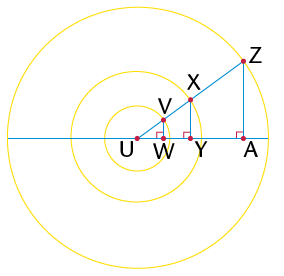• Use a protractor to measure the interior angles of each of the following triangles. Write the sizes of the angles on the diagrams.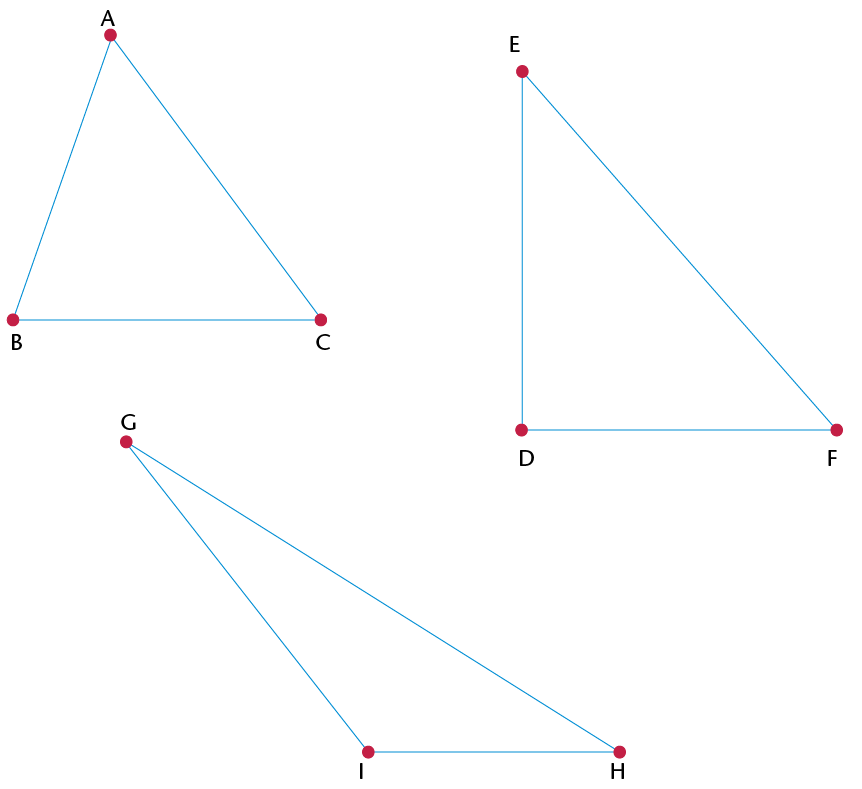• Classify the triangles in question 1 according to their angle properties. Choose from the following types of triangles: acute-angled, obtuse-angled and right-angled.
1. $\triangle$ABC is an _______ triangle, because
2. $\triangle$EDF is a _______ triangle, because
3. $\triangle$GHI is an obtuse-angled triangle, because
• The marked angles in each triangle below are equal. Classify the triangles according to angle and side properties.
1. $\triangle$ .......... is an acute isosceles triangle, because ........ and .........
2. $\triangle$ .......... is a right-angled isosceles triangle, because ......... and ........
3. $\triangle$ .......... is an obtuse isosceles triangle, because ........... and ..........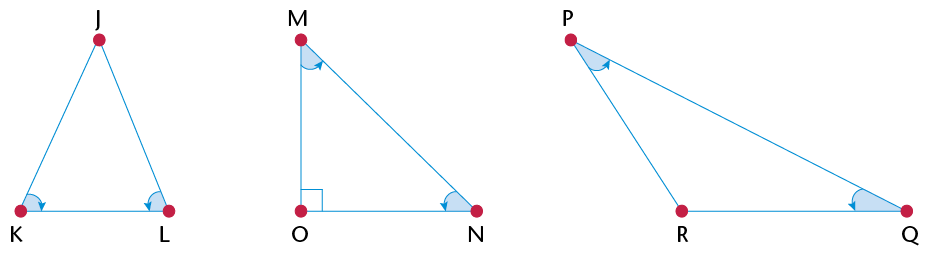• Say for what kind of triangle each statement is true. If it is true for all triangles, then write 'All triangles'.
 Statement True for (a) Two sides of the triangle are equal. (b) One angle of the triangle is obtuse. (c) Two angles of the triangle are equal. (d) All three angles of the triangle are equal to 60$^\circ$. (e) The size of an exterior angle is equal to the sum of the opposite interior angles. (f) The longest side of the triangle is opposite the biggest angle. (g) The sum of the two shorter sides of the triangle is bigger than the length of the longest side. (h) The square of the length of one side is equal to the sum of the squares of the other sides. (i) The square of the length of one side is bigger than the sum of the squares of the other sides. (j) The sum of the interior angles of the triangle is 180$^\circ$.
•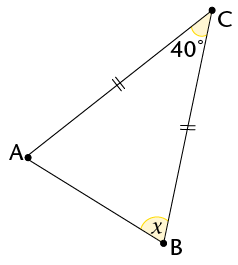Statement Reason $\text{AC} = \text{BC}$ Given $\therefore\hat{\text{A}} = \hat{\text{B}}$ $180^\circ = 40^\circ + x + x$ Sum $\angle$s $\triangle$ $180^\circ = 40^\circ + 2x$ $\therefore x =$
• Calculate the sizes of the unknown angles.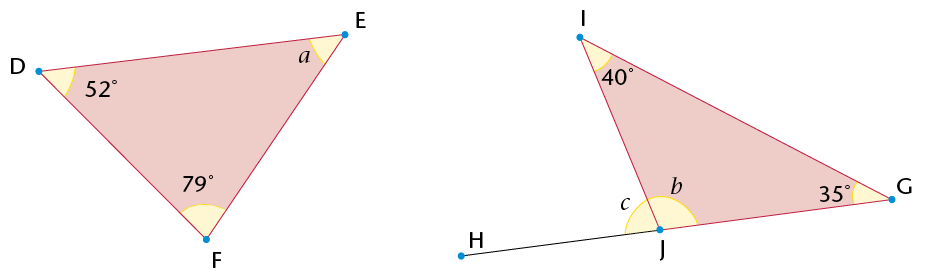• Determine the sizes of the unknown angles and the length of MO.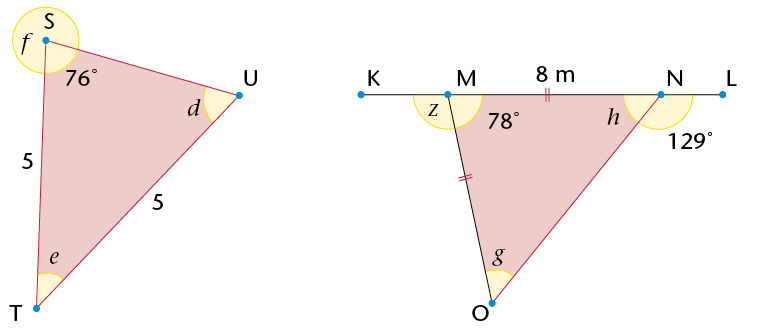• Calculate the sizes of y and x.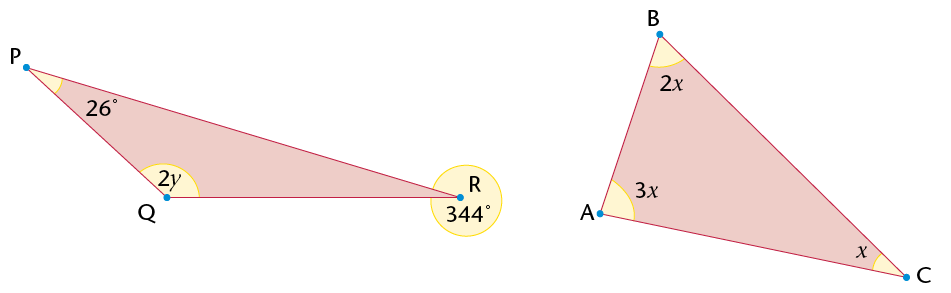• Name the following quadrilaterals. Mark equal angles and equal sides in each figure. Use your ruler and protractor to measure angle sizes and lengths where necessary.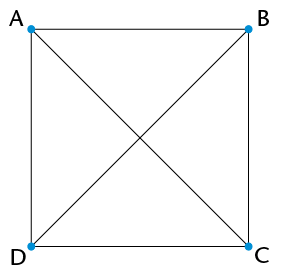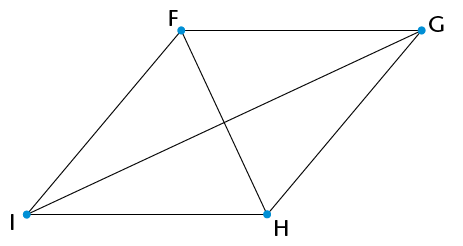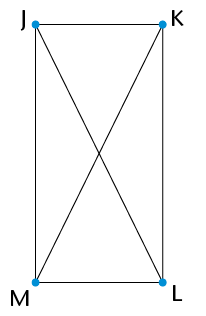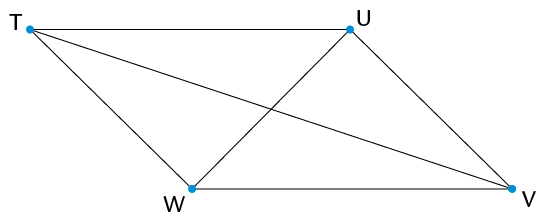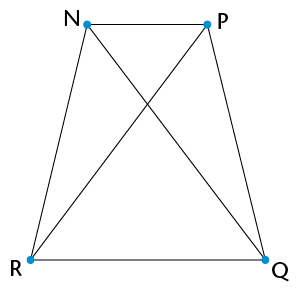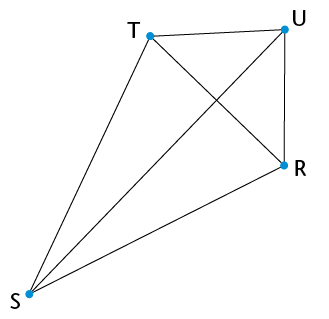• Complete the following table:
 Properties True for the following quadrilaterals Square Rhombus Rectangle Parallelogram Kite Trapezium At least one pair of opposite angles is equal. yes yes yes yes yes no Both pairs of opposite angles are equal. At least one pair of adjacent angles is equal. All four angles are equal. Any two opposite sides are equal. Two adjacent sides are equal, and the other two adjacent sides are also equal. All four sides are equal. At least one pair of opposite sides is parallel. Any two opposite sides are parallel. The two diagonals are perpendicular. At least one diagonal bisects the other one. The two diagonals bisect each other. The two diagonals are equal. At least one diagonal bisects a pair of opposite angles. Both diagonals bisect a pair of opposite angles. The sum of the interior angles is 360$^\circ$.
• Look at the properties of a square and a rhombus.
1. Are all the properties of a square also the properties of a rhombus? Explain.
2. Are all the properties of a rhombus also the properties of a square? Explain.
3. Which statement is true?

A square is a special kind of rhombus.

A rhombus is a special kind of square.

• Look at the properties of a square and a rectangle.
1. Are all the properties of a square also the properties of a rectangle? Explain.
2. Are all the properties of a rectangle also the properties of a square? Explain.
3. Which statement is true?

A square is a special kind of rectangle.

A rectangle is a special kind of square.

• Look at the properties of a parallelogram and a rectangle.
1. Are all the properties of a parallelogram also the properties of a rectangle? Explain.
2. Are all the properties of a rectangle also the properties of a parallelogram? Explain.
3. Which statement is true?

A parallelogram is a special kind of rectangle.

A rectangle is a special kind of parallelogram.

• Look at the properties of a rhombus and a parallelogram. Is a rhombus a special kind of parallelogram? Explain.
• Compare the properties of a kite and a parallelogram. Why is a kite not a special kind of parallelogram?
• Compare the properties of a trapezium and a parallelogram. Why is a trapezium not a special kind of parallelogram?
• Determine the sizes of angles a to e in the quadrilaterals below. Give reasons for your answers.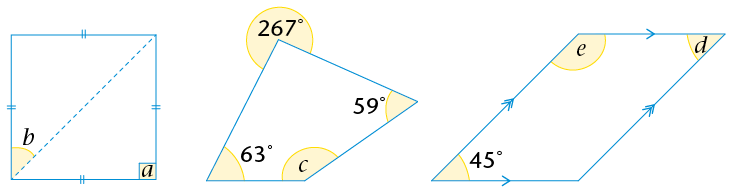• Calculate the perimeters of the quadrilaterals on the right. Give your answers to two decimal places.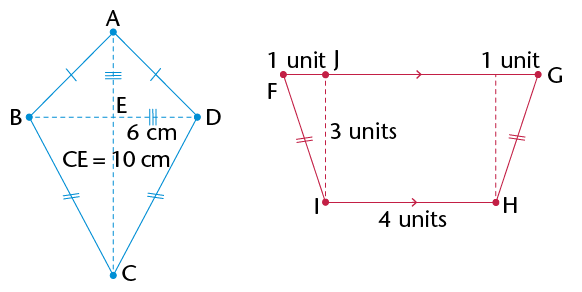• words, if you cut out one of the triangles and place it on the other, they will match exactly.

If you know that two triangles are congruent, then each side in the one triangle will be equal to each corresponding side in the second triangle. Also, each angle in the one triangle will be equal to each corresponding angle in the second triangle.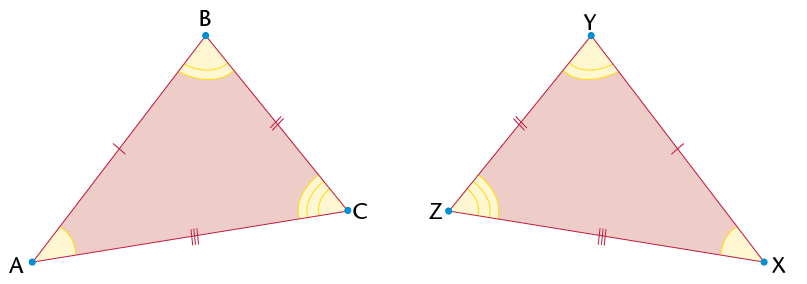$\equiv$ $\triangle$XYZ.

Congruency symbol

$\equiv$ means 'is congruent to'

• $\triangle$KLM $\equiv$ $\triangle$PQR:
• $\triangle$FGH $\equiv$ $\triangle$CST:
• The conditions for congruency:

• SSS (all corresponding sides are equal)
• SAS (two corresponding sides and the angle between the two sides are equal)
• AAS (two corresponding angles and any corresponding side are equal)
• RHS (both triangles have a $90^\circ$ angle and have equal hypotenuses and one other side equal)
•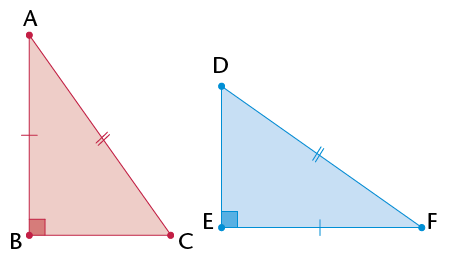•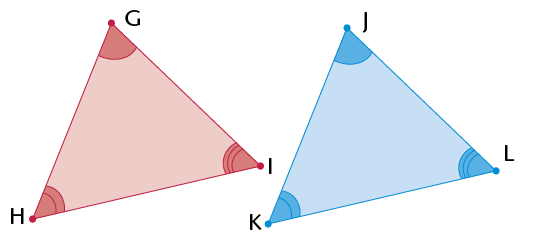•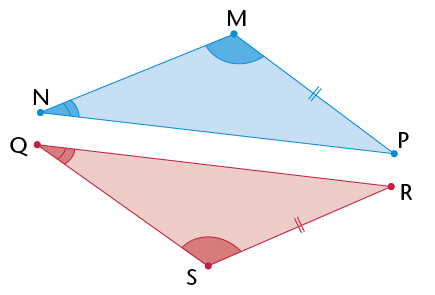•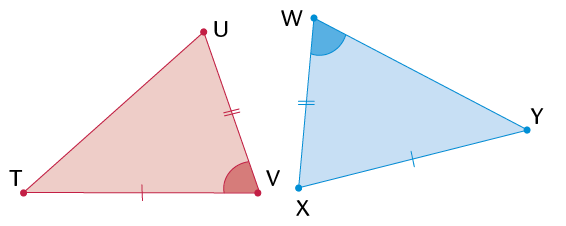•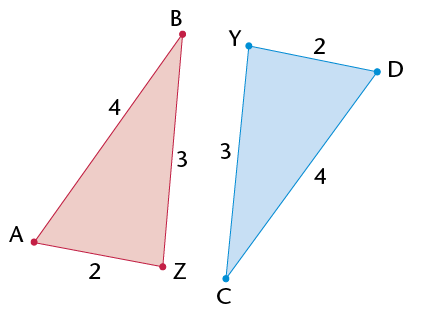•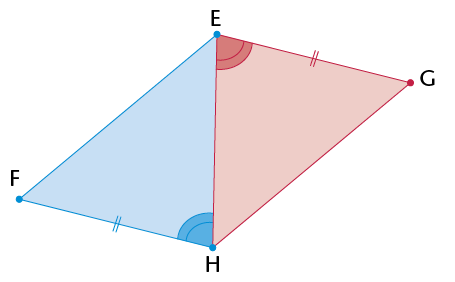• Each statement you make needs a reason.
• You must give three statements to prove any two triangles congruent.
• Give the reason for congruency.
Example: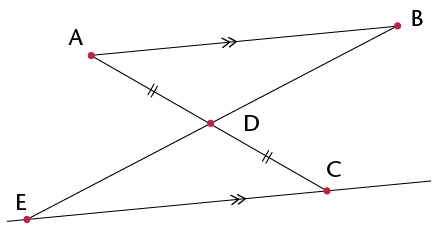Solution:

Statement

Reason

In $\triangle$ABD and $\triangle$CED:

2) $\text{A}\hat{\text{D}}\text{B} = \text{C}\hat{\text{D}}\text{E}$

3) $\text{B}\hat{\text{A}}\text{D} = \text{E}\hat{\text{C}}\text{D}$

$\therefore$ $\triangle$ABD $\equiv$ $\triangle$CED

Given

Vert. opp. $\angle$s

Alt. $\angle$s (AB$||$ EC)

AAS

• Prove that $\triangle$ACE $\equiv$ $\triangle$BDE.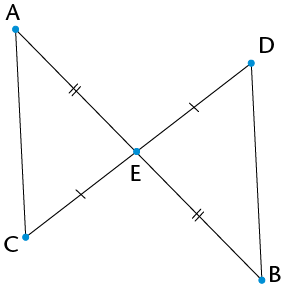Statement Reason
• Prove that $\triangle$WXZ $\equiv$ $\triangle$YXZ.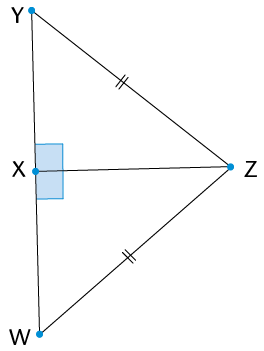Statement Reason
• Prove that QR = SP. (Hint: First prove that the triangles are congruent.)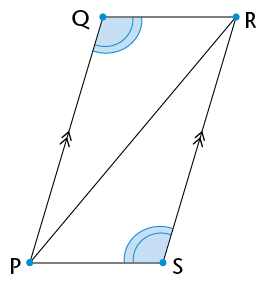Statement Reason
• Prove that the triangles below are congruent. Then find the size of $\text{Q}\hat{\text{M}}\text{P}$.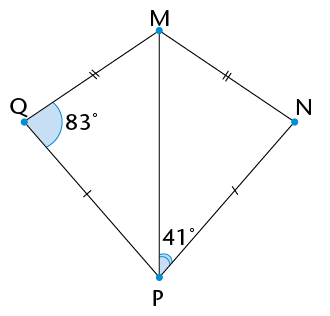Statement Reason
•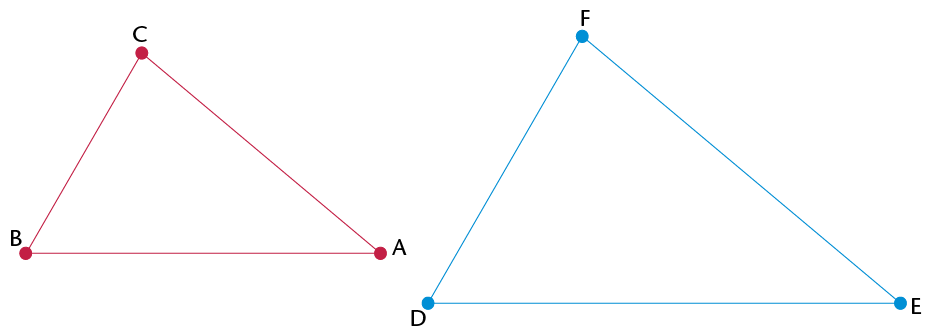1. Use a protractor to measure the angles in each triangle above. Then complete the table below.
 Angle Angle What do you notice? $\hat{\text{B}} =\quad$ $\hat{\text{D}} =$ $\hat{\text{A}} =$ $\hat{\text{E}} =$ $\hat{\text{C}} =$> $\hat{\text{F}} =\quad$
2. What can you say about the sizes of the angles in similar triangles?
1. Use a ruler to measure the lengths of the sides in each triangle in question 1. Then complete the table below.
 Length (cm) Length (cm) Ratio BA = DE = BA : DE = $\quad$ = $1 : 1\frac{1}{3}$ BC = DF = BC : DF = $\quad$ = $\quad$ CA = FE = CA : FE = $\quad$ = $\quad$
2. What can you say about the relationship between the sides in similar triangles?

Ratio reminder

You read 2 : 1 as 'two to one'.

1. The following notation shows that the triangles are similar: $\triangle$BAC $|||$ $\triangle$DEF. Why do you think we write the first triangle as $\triangle$BAC and not as $\triangle$ABC?

The properties of similar triangles:

• The corresponding angles are equal.
• The corresponding sides are in proportion.

Notation for similar triangles:

If $\triangle$XYZ is similar to $\triangle$PQR, then we write: $\triangle$XYZ $|||$ $\triangle$PQR.

Angles: $\hat{\text{X}} = \hat{\text{P}}$ and $\hat{\text{Y}} = \hat{\text{Q}}$ and $\hat{\text{Z}} = \hat{\text{R}}$

Sides: $\text{X}$$\text{Y}$:$\text{P}$$\text{Q}=\text{X}$$\text{Z}$: $\text{P}$$\text{R}=\text{Y}$$\text{Z}$:$\text{Q}$$\text{R}$

When proving that triangles are similar, you either need to show that the corresponding angles are equal or you must show that the sides are in proportion.

• Decide if the following triangles are similar to each other.
1.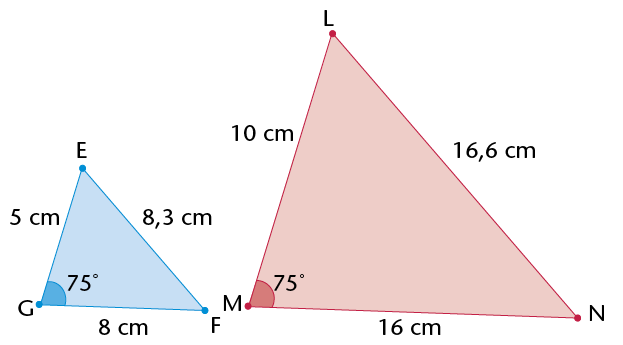2.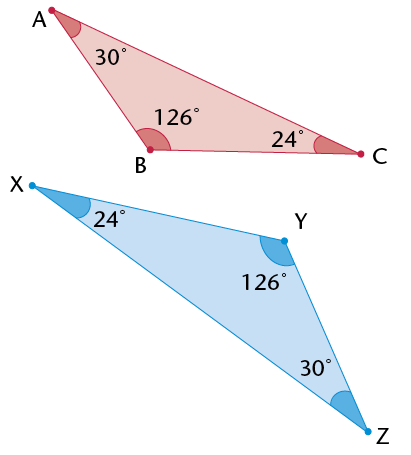3.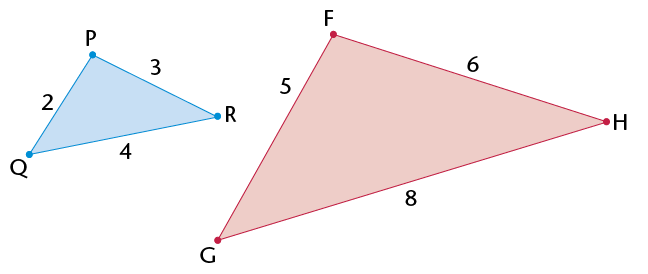4.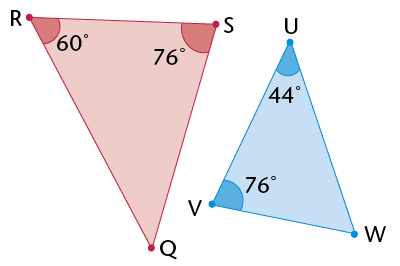• Use a ruler and protractor to construct the triangles described in (a) to (d) below.
• Use your knowledge of similarity to draw the second triangle in each question.
• Indicate the sizes of the corresponding sides and angles on the second triangle.
1. In $\triangle$EFG, $\hat{\text{G}}$= 75$^\circ$, EG = 4 cm and GF = 5 cm. $\triangle$ABC is an enlargement of $\triangle$EFG, with its sides three times longer.
2. In $\triangle$MNO, $\hat{\text{M}}$= 45$^\circ$, $\hat{\text{N}}$= 30$^\circ$ and MN = 5 cm. $\triangle$PQR is similar to $\triangle$MNO. The sides of $\triangle$MNO to $\triangle$PQR are in proportion 1 : 3.
3. $\triangle$RST is an isosceles triangle. $\hat{\text{R}}$= 40$^\circ$, RS is 10 cm and RS = RT. $\triangle$VWX is similar to $\triangle$RST. The sides of $\triangle$RST to $\triangle$VWX are in proportion 1:$\frac{1}{2}$.
4. $\triangle$KLM is right-angled at $\hat{\text{L}}$, LM is 7 cm and the hypotenuse is 12 cm. $\triangle$XYZ is similar to $\triangle$KLM, so that the sides are a third of the length of $\triangle$KLM.
1. Which of the following are minimum conditions for similar triangles?

1. Two angles in one triangle are equal to two angles in another triangle.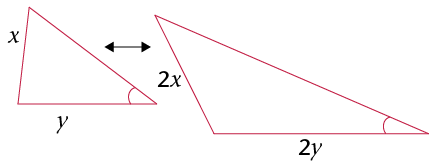2. Two sides of one triangle are in the same proportion as two sides in another triangle.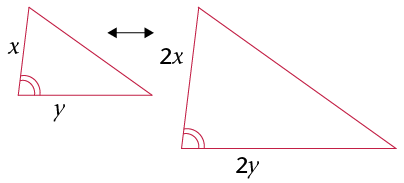3. Two sides of one triangle are in the same proportion as two sides in another triangle, and the angle between the two sides is equal to the angle between the corresponding sides.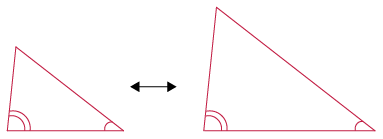4. Two sides of one triangle are in the same proportion as two sides in another triangle, and one angle not between the two sides is equal to the corresponding angle in the other triangle.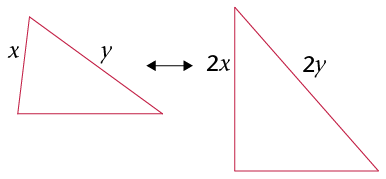Parallel lines never meet. Two lines are parallel to each other if the distance between them is the same along the whole length of the lines.

• Line segment QR is parallel to line segment ST.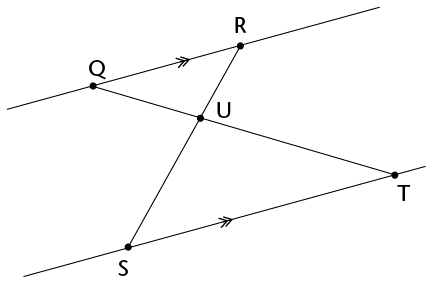Complete the following proof that $\triangle$QRU $|||$ $\triangle$TSU:

 Statement Reason $\text{R}$$\hat{\text{Q}}$$\text{T}$ = $\text{Q}$$\hat{\text{T}}$$\text{S}$ $\text{Q}$$\hat{\text{R}}$$\text{S}$=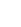= $\therefore$$\triangle$QRU$|||$ $\triangle$TSU Alt. $\angle$s Vert. opp. $\angle$s Equal $\angle$s (or AAA)
• The following intersecting line segments form triangle pairs between parallel lines.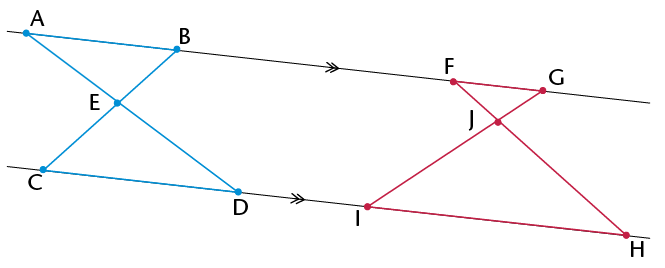1. Are the triangles in each pair similar? Explain.
2. Write down pairs of similar triangles.
3. Are triangles like these always similar? Explain how you can be sure without measuring every possible triangle pair.
• The intersecting lines on the right form triangle pairs between the line segments that are not parallel. Are these triangle pairs similar? Explain why or why not.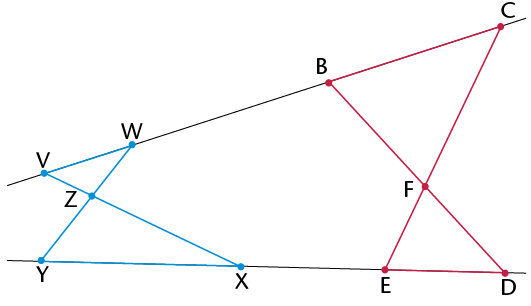• Consider the triangles below. DE$||$ BC. Prove that $\triangle$ABC$|||$ $\triangle$ADE.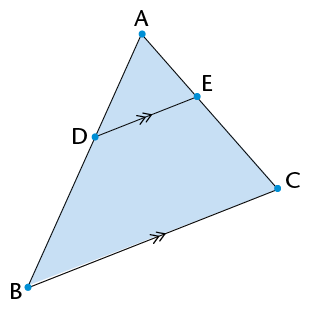Statement Reason
• In the diagram on the right, ST is a telephone pole and UV is a vertical stick. The stick is 1 m high and it casts a shadow of 1,7 m (VW). The telephone pole casts a shadow of 5,1 m (TW). Use similar triangles to calculate the height of the telephone pole.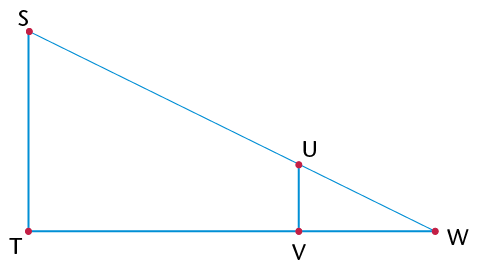• How many similar triangles are there in the diagram below? Explain your answer.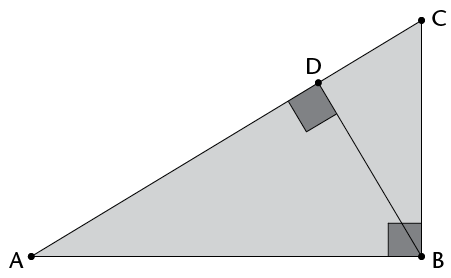• $\triangle$ABC on the right is equilateral. D is the midpoint of AB, E is the midpoint of BCand F is the midpoint of AC.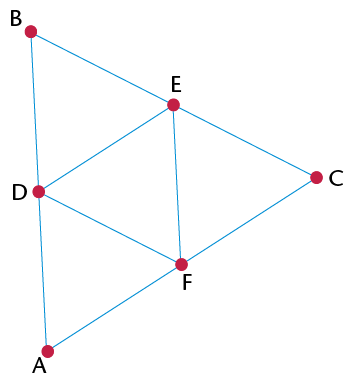1. Prove that $\triangle$BDE is an equilateral triangle.
2. Find all the congruent triangles. Give a proof for each.
3. Name as many similar triangles as you can. Explain how you know they are similar.
4. What is the proportion of the corresponding sides of the similar triangles?
5. Prove that DE is parallel to AC.
6. Is DF parallel to BC? Is EF parallel to BA? Explain.
• Consider the similar triangles drawn below using concentric circles. Explain why the triangles are similar in each diagram.
1.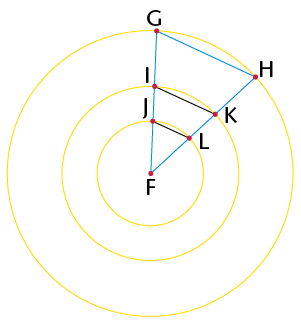2.